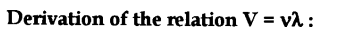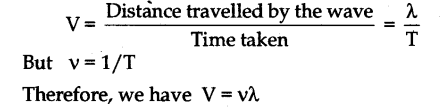# Define the following terms and state their SI units : (a) Wavelength (b) Time period (c) Amplitude. Establish the relation V = vk, where the symbols have their usual meanings

(a) Wavelength : It is defined as the distance travelled by a wave during the time a particle of the medium completes one vibration. It is denoted by k. Its SI unit is metre.
(b) Time period : It is defined as the time by a vibrating particle to complete one vibration. It is denoted by T. Its SI unit is second.
© Amplitude : It is defined as the maximum displacement produced in the vibrating particle on either side of the mean position. It is denoted by A. Its SI unit is metre.

We know that, wavelength k is the distance covered by the wave during the time a particle of the medium completes one vibration i.e., T.